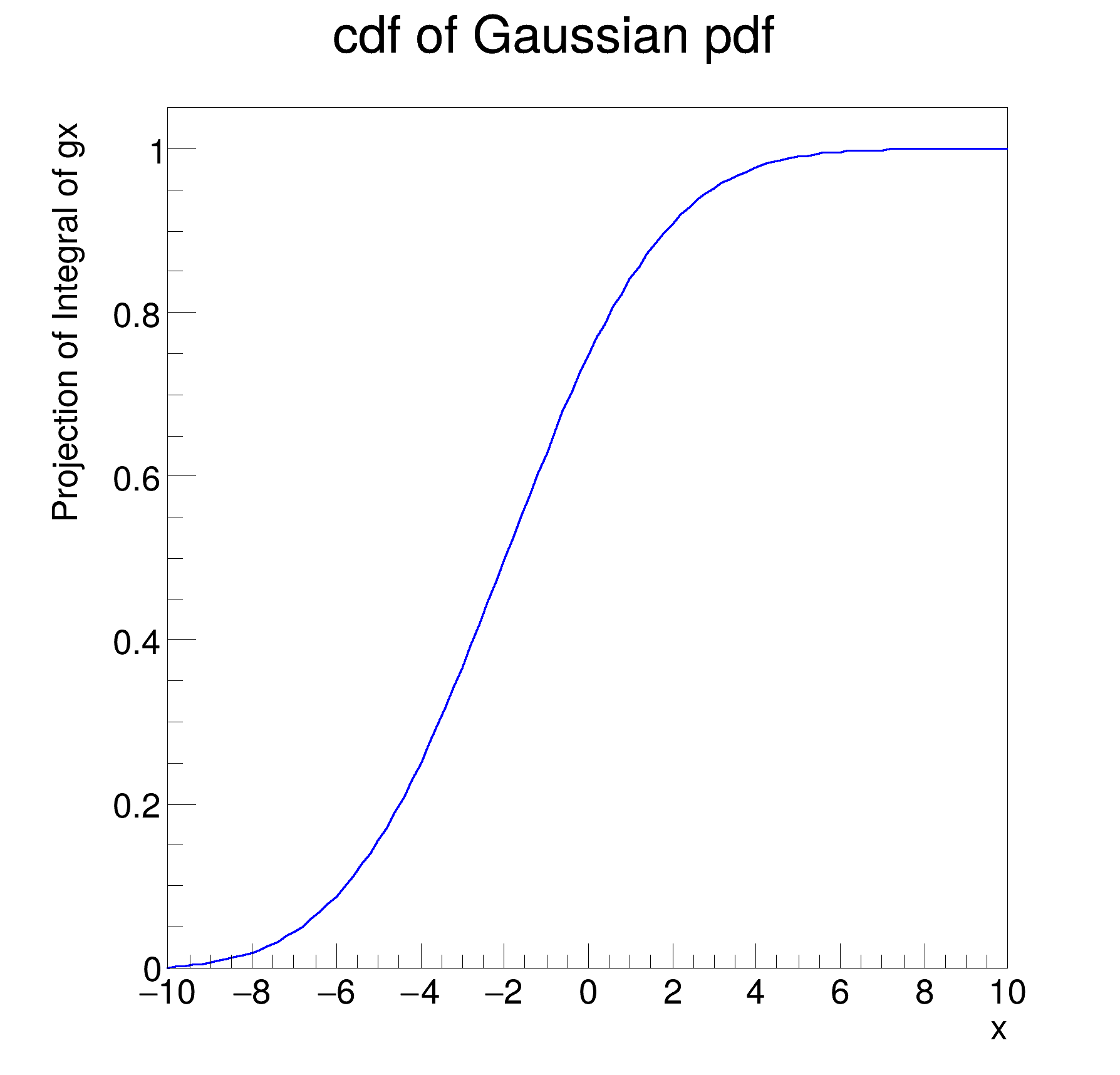ROOT   Reference Guide
Searching...
No Matches
rf110_normintegration.C File Reference

## Detailed DescriptionBasic functionality: normalization and integration of pdfs, construction of cumulative distribution monodimensional functions#include "RooRealVar.h"
#include "RooGaussian.h"
#include "RooAbsReal.h"
#include "RooPlot.h"
#include "TCanvas.h"
#include "TAxis.h"
using namespace RooFit;
{
// S e t u p m o d e l
// ---------------------
// Create observables x,y
RooRealVar x("x", "x", -10, 10);
// Create pdf gaussx(x,-2,3)
RooGaussian gx("gx", "gx", x, -2.0, 3.0);
// R e t r i e v e r a w & n o r m a l i z e d v a l u e s o f R o o F i t p . d . f . s
// --------------------------------------------------------------------------------------------------
// Return 'raw' unnormalized value of gx
cout << "gx = " << gx.getVal() << endl;
// Return value of gx normalized over x in range [-10,10]
RooArgSet nset(x);
cout << "gx_Norm[x] = " << gx.getVal(&nset) << endl;
// Create object representing integral over gx
// which is used to calculate gx_Norm[x] == gx / gx_Int[x]
std::unique_ptr<RooAbsReal> igx{gx.createIntegral(x)};
cout << "gx_Int[x] = " << igx->getVal() << endl;
// I n t e g r a t e n o r m a l i z e d p d f o v e r s u b r a n g e
// ----------------------------------------------------------------------------
// Define a range named "signal" in x from -5,5
x.setRange("signal", -5, 5);
// Create an integral of gx_Norm[x] over x in range "signal"
// This is the fraction of of pdf gx_Norm[x] which is in the
// range named "signal"
std::unique_ptr<RooAbsReal> igx_sig{gx.createIntegral(x, NormSet(x), Range("signal"))};
cout << "gx_Int[x|signal]_Norm[x] = " << igx_sig->getVal() << endl;
// C o n s t r u c t c u m u l a t i v e d i s t r i b u t i o n f u n c t i o n f r o m p d f
// -----------------------------------------------------------------------------------------------------
// Create the cumulative distribution function of gx
// i.e. calculate Int[-10,x] gx(x') dx'
std::unique_ptr<RooAbsReal> gx_cdf{gx.createCdf(x)};
// Plot cdf of gx versus x
RooPlot *frame = x.frame(Title("cdf of Gaussian pdf"));
gx_cdf->plotOn(frame);
// Draw plot on canvas
new TCanvas("rf110_normintegration", "rf110_normintegration", 600, 600);
frame->GetYaxis()->SetTitleOffset(1.6);
frame->Draw();
}
RooArgSet is a container object that can hold multiple RooAbsArg objects.
Definition RooArgSet.h:55
Plain Gaussian p.d.f.
Definition RooGaussian.h:24
A RooPlot is a plot frame and a container for graphics objects within that frame.
Definition RooPlot.h:43
static RooPlot * frame(const RooAbsRealLValue &var, double xmin, double xmax, Int_t nBins)
Create a new frame for a given variable in x.
Definition RooPlot.cxx:239
TAxis * GetYaxis() const
Definition RooPlot.cxx:1279
void Draw(Option_t *options=nullptr) override
Draw this plot and all of the elements it contains.
Definition RooPlot.cxx:652
RooRealVar represents a variable that can be changed from the outside.
Definition RooRealVar.h:37
virtual void SetTitleOffset(Float_t offset=1)
Set distance between the axis and the axis title.
Definition TAttAxis.cxx:298
The Canvas class.
Definition TCanvas.h:23
Double_t x[n]
Definition legend1.C:17
The namespace RooFit contains mostly switches that change the behaviour of functions of PDFs (or othe...
const char * Title
Definition TXMLSetup.cxx:68
Ta Range(0, 0, 1, 1)
gx = 0.800737
gx_Norm[x] = 0.106896
gx_Int[x] = 7.49084
[#1] INFO:Eval -- RooRealVar::setRange(x) new range named 'signal' created with bounds [-5,5]
gx_Int[x|signal]_Norm[x] = 0.834753
Date
July 2008

Definition in file rf110_normintegration.C.